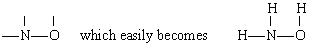Deciding on a Skeleton Structure

Back to Writing Lewis Structures for Molecules

The skeleton structure of a covalent molecule can often be determined by considering the valences of the constituent atoms. Usually the atom which forms the largest number of bonds is found in the center of the skeleton, where it can connect to the maximum number of other atoms.

Example 1: Hypochlorous acid has the molecular formula HOCl. Draw a

structural formula.

Solution There are several possible ways to link the atoms togetherThe usual valence of H is 1, and so structures 3 and 4, which have two bonds to H, may be eliminated. The usual valence of Cl is also 1, and so structure 2 may also be ruled out. Structure 1 shows H forming one bond, Cl forming one, and O forming two, in agreement with the usual valences, and so it is chosen.

The total number of valence electrons available is 1 from H plus 6 from O plus 7 from Cl, or 14. Filling these into the skeleton we haveNote that O, which had the largest valence, is in the center of the skeleton.

Example 2:

Draw a structural formula for hydroxylamine, NH3O.

Solution In this case N has the largest valence (3), followed by O (2) and H (1). Both N and O can form “bridges” between other atoms, but H cannot. Therefore we place N and O in the center of the skeleton to giveby addition of the three H atoms.

There are a total of 5 + 3 + 6 = 14 valence electrons from N, 3H’s and O. These can be placed as follows:Once the Lewis diagram has been determined, the molecular formula is often rewritten to remind us of what the structural formula is. For example, the molecular formula for hydroxylamine is usually written NH2OH instead of NH3O to remind us that two H’s are bonded to N and one to O. It is assumed that the person reading the formula will realize that N and O each have one valence electron left to share with each other, connecting —NH2 with —OH. In some cases more than one skeleton structure will satisfy the valence of each atom and the octet rule as well. For example, you can verify that the molecular formula C2H6O corresponds to both of the following:In such a case we can only decide which molecular structure we have by experiment. The properties of ethyl alcohol when diluted with water and consumed are well known. Dimethyl ether is a gas. Like the diethyl ether used in operating rooms, it is highly explosive and can put you to sleep. Two molecules, such as dimethyl ether and ethyl alcohol, which have the same molecular formula but different structural formulas are said to be isomers.